# NCERT solutions class 9 maths chapter 7 Exercise 7.1

In this page we have NCERT solutions class 9 maths chapter 7 Triangles for EXERCISE 7.1 . Hope you like them and do not forget to like , social share and comment at the end of the page.
We must revise these notes before proceding with the questions

## Different Criterion for Congruence of the triangles

### SAS Congurence (AXIOM)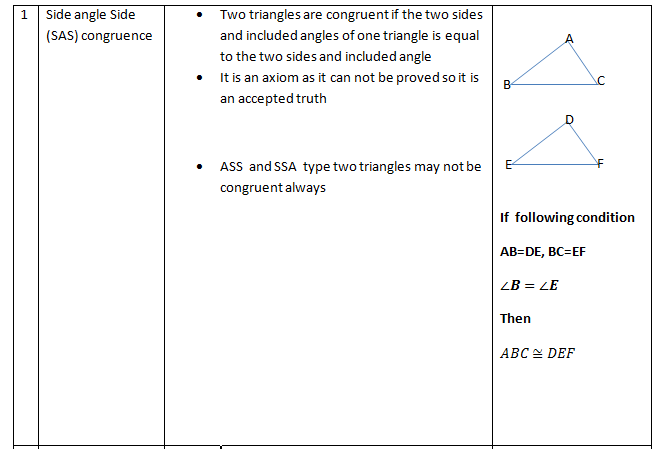### ASA Congurence (Theorem)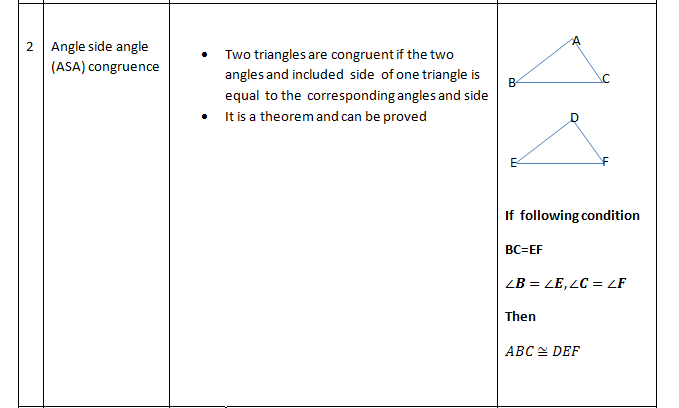### AAS Congurence (Theorem)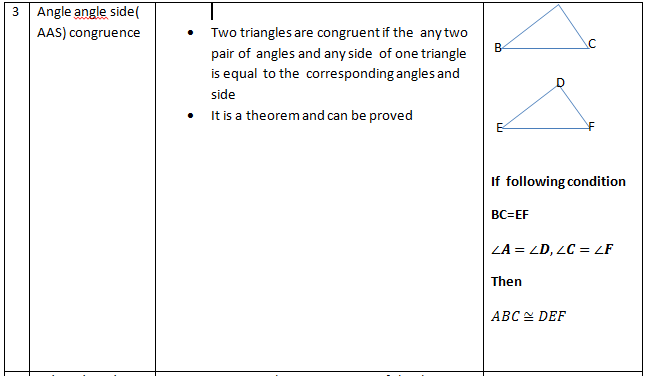### SSS Congurence (Theorem)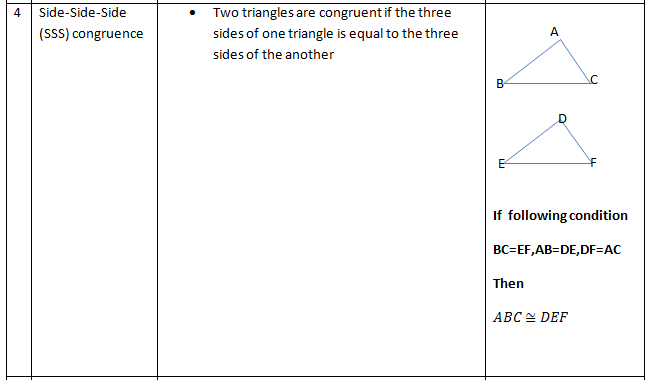### RHS Congurence (Theorem)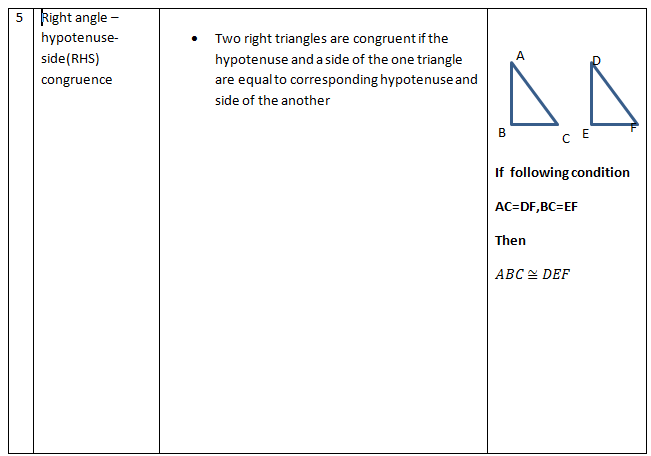Caveat
AAA( Angle Angle Angle) is not the right condition to Prove congurence.
How to Prove the congurence of Two Triangle
1) We have already studied that two triangles are congurent when all the sides and all the angles are equal. But we dont need prove all these while solving the Problem
2) We just need to prove the congurence using the different criterio like SSS,ASA,SAS,RHS,AAS
3) Dont use AAA
4) Use the theorem learn in previous Geometry chapter like vertically opposite angles,alternate interior angles,corresonding angles
5)Write down the corresponding angles and corresponding sides carefully
6) We need to be careful with the labelling when our Triangles are in different positions

Question 1
In quadrilateral ACBD, AC = AD and AB bisects ∠A (see below figure). Show that ΔABC ≅ ΔABD. What can you say about BC and BD?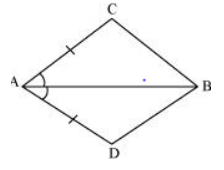Given,
AC = AD and AB bisects ∠A
To prove,
ΔABC ≅ ΔABD
Proof, In ΔABC and ΔABD,
AB = AB (Common)
∠CAB = ∠DAB (AB is bisector)

By SAS (Side-Angle-Side) congruence condition.
Therefore, ΔABC ≅ ΔABD.

Now from CPCT, we know that

BC=BD

Question 2
ABCD is a quadrilateral in which AD = BC and ∠DAB = ∠CBA (see Fig. 7.17). Prove that
(i) ΔABD ≅ ΔBAC
(ii) BD = AC
(iii) ∠ABD = ∠BAC.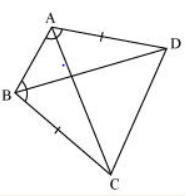Given,
AD = BC and ∠DAB = ∠CBA

(i) In ΔABD and ΔBAC,
AB = BA (Common)
∠DAB = ∠CBA (Given) AD = BC (Given)

By SAS congruence condition.
So, ΔABD ≅ ΔBAC
(ii) Since, ΔABD ≅ ΔBAC
Therefore BD = AC by CPCT
(iii) Since, ΔABD ≅ ΔBAC
Therefore ∠ABD = ∠BAC by CPCT

Question 3
AD and BC are equal perpendiculars to a line segment AB (see below figure). Show that CD bisects AB.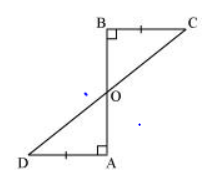Given,
AD and BC are equal perpendiculars to AB.
To prove,
CD bisects AB
Proof,
In ΔAOD and ΔBOC,
∠A = ∠B (As Perpendicular)
∠AOD = ∠BOC (Vertically opposite angles)

By AAS(Angle-Angle-Side) congruence condition.

So, ΔAOD ≅ ΔBOC

Now by CPCT
AO = OB

So  CD bisects AB.

Question 4
l and m are two parallel lines intersected by another pair of parallel lines p and q (see below figure). Show that ΔABC ≅ ΔCDA.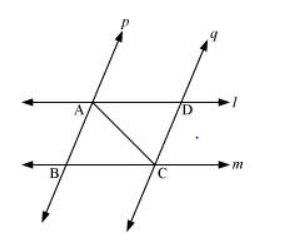Given,
l || m and p || q
To prove,
ΔABC ≅ ΔCDA
Proof,
In ΔABC and ΔCDA,
∠BCA = ∠DAC (Alternate interior angles) AC = CA (Common)
∠BAC = ∠DCA (Alternate interior angles)

By ASA(Angle-Side-Angle) congruence condition. So, ΔABC ≅ ΔCDA

Question 5
Line l is the bisector of an angle ∠A and B is any point on l. BP and BQ are perpendiculars from B to the arms of ∠A (see below figure). Show that:
(i) ΔAPB ≅ ΔAQB
(ii) BP = BQ or B is equidistant from the arms of ∠A.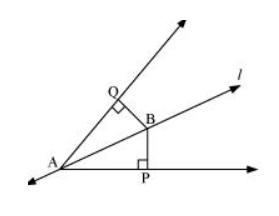Given,
l is the bisector of an angle ∠A.
BP and BQ are perpendiculars.

(i) In ΔAPB and ΔAQB,
∠P = ∠Q (Right angles)
∠BAP = ∠BAQ (l is bisector)
AB = AB (Common)
Therefore, ΔAPB ≅ ΔAQB by AAS congruence condition.
(ii) BP = BQ by CPCT. Therefore, B is equidistant from the arms of ∠A.

Question 6
In below figure, AC = AE, AB = AD and ∠BAD = ∠EAC. Show that BC = DE.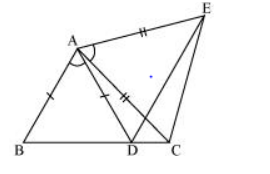Given,
To Prove,
BC = DE
Proof,

AC = AE (Given)
By SAS (Side -Angle-Side) congruence condition.

By CPCT

BC = DE

Question 7
AB is a line segment and P is its mid-point. D and E are points on the same side of AB such that ∠BAD = ∠ABE and ∠EPA = ∠DPB (see below figure). Show that
(i) ΔDAP ≅ ΔEBP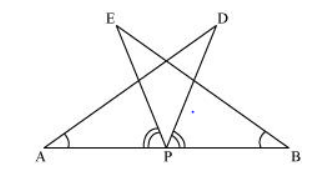Given,
P is mid-point of AB.
∠BAD = ∠ABE and ∠EPA = ∠DPB

(i) ∠EPA = ∠DPB

∠EPA + ∠DPE = ∠DPB + ∠DPE ∠DPA = ∠EPB
In ΔDAP ≅ ΔEBP,
∠DPA = ∠EPB
AP = BP (P is mid-point of AB)

By ASA congruence condition. So, ΔDAP ≅ ΔEBP
(ii) By CPCT

Question 8
In right triangle ABC, right angled at C, M is the mid-point of hypotenuse AB. C is joined to M and produced to a point D such that DM = CM. Point D is joined to point B (see below figure). Show that:
(i) ΔAMC ≅ ΔBMD
(ii) ∠DBC is a right angle.
(iii) ΔDBC ≅ ΔACB
(iv) CM = 1/2 AB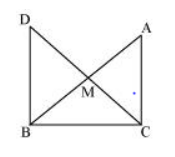Given,
∠C = 90°, M is the mid-point of AB and DM = CM

(i) In ΔAMC and ΔBMD,
AM = BM (M is the mid-point)
∠CMA = ∠DMB (Vertically opposite angles)
CM = DM (Given)
By SAS(Side-Angle-Side) congruence condition
So, ΔAMC ≅ ΔBMD.

(ii)By CPCT

∠ACM = ∠BDM
Therefore, AC || BD as alternate interior angles are equal.
Now,
∠ACB + ∠DBC = 180° (co-interiors angles)
90° + ∠B = 180°
∠DBC = 90°
(iii) In ΔDBC and ΔACB,
BC = CB (Common)
∠ACB = ∠DBC (Right angles)
DB = AC (by CPCT, already proved)

By (Side-Angle-Side) congruence condition.
So, ΔDBC ≅ ΔACB

(iv)  DC = AB (ΔDBC ≅ ΔACB)
DM = CM = AM = BM (M is mid-point)
DM + CM = AM + BM CM + CM = AB

CM = 1/2AB

## Summary

1. NCERT solutions class 9 maths chapter 7 Triangles Exercise 7.1 has been prepared by Expert with utmost care. If you find any mistake.Please do provide feedback on mail. You can download the solutions as PDF in the below Link also
2. This chapter 7 has total 3 Exercise 7.1 ,7.2 and 7.3. This is the First exercise in the chapter.